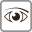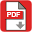## Abstract

In this paper, we propose a robust high-dimensional data reduction method. The model assumes that the pixel reflec-tance results from linear combinations of pure component spectra contaminated by an additive noise. The abundance parameters appearing in this model satisfy positivity and additive constraints. These constraints are naturally expressed in a Bayesian literature by using appropriate abundance prior distributions. The posterior distributions of the unknown model parameters are then derived. The proposed algorithm consists of Bayesian inductive cognition part and hierarchical reduction algorithm model part. The pro-posed reduction algorithm based on Bayesian inductive cognitive model is used to decide which dimensions are advantageous and to output the recommended dimensions of the hyperspectral image. The algorithm can be interpreted as a robust reduction inference method for a Bayesian inductive cognitive model. Experimental results on high-dimensional data demonstrate useful properties of the proposed reduction algorithm.

# AttachmentsViews: 62PDF: 30